## Solving Laplace’s equation given a surface potential

Interesting problem appeared recently. It’s equivalent to solving the electrostatic potential above a plane given the surface potential on that plane.

Say the plane is at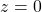and we wish to find the electrostatic potential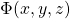for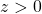given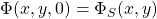. We start with the divergence theorem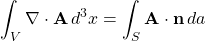where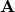is a well behaved vector field defined in the volume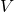bounded by the closed surface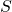. Let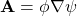where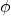and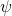are arbitrary scalar fields. Then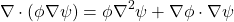and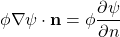where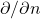is the normal derivative at the surface(directed outwards from inside the volume). Substituting the above equations back into the divergence equation, we find Green’s first identity: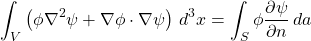Interchangingandin the above and subtracting the two equations (the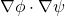term cancels) we obtain Green’s theorem (or Green’s second identity):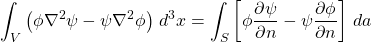.

We now choose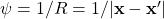where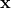is the observation point and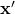is the integration variable and let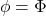, the electrostatic potential. Now use the facts that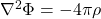and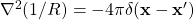and we find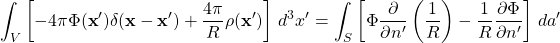Iflies within the volume, we obtain: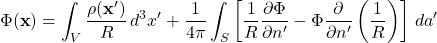Iflies outside ofthen the left hand side of the above equation is 0.

This equation is not a solution of the boundary value problem but is simply an integral statement since the right hand side is an overspecification of the problem. We can define a Green’s function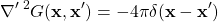where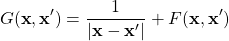and the function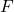satisfies Laplace equation inside the volume: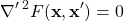We see if we use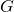and the freedom thatallows us, we can now use Green’s theorem to eliminate one or the other surface terms. If we take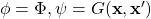and plug this back into Green’s theorem we find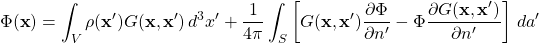Since we are interested in the Dirichlet boundary condition (that is we are given the potential on), we demand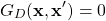foron. Then we have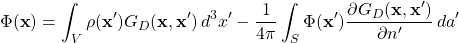If one is interested in the Neuman boundary condition (that is we have the normal component of the field on), then we can define the corresponding Green’s function: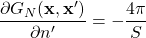foron. Similarly as before, we can use Green’s theorem to find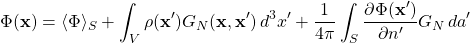where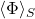is the average value of the potential over the surface- typically an irrelevant constant.

For the Dirichlet case, it can be shown via Green’s theorem, that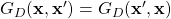while for the Neuman case,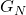can be forced to have the same property.

In our problem,consists of the upper half infinite volume. If we assume that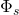falls to zero at large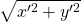the surface integral only survives for. Since we are specifying the potential on theplane we need to find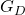. By the method of images, we can immediately write it down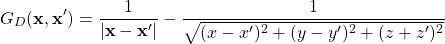We see that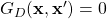when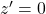as required. We also note that the second term on the right hand side does satisfy Laplace’s equation in. Therefore by uniqueness we have the correct Green’s function. We next need to compute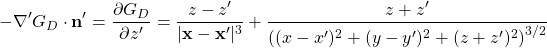Plugging this back into the equation for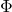and assuming there are no charges in the volume (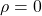) we find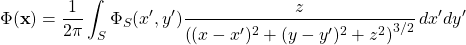This is the solution to our problem. We see that given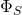we have an integral equation to findfor. Furthermore, we see the Green’s function only depends on the differences of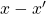and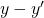, or equivalently,is simply a 2D convolution ofand the kernel (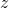being viewed as simply a parameter for the surface integral to determine where inthe potential should be evaluated at).

If one is more interested in the electrostatic field (vs. potential), we use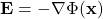and we can find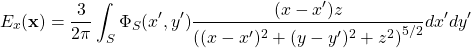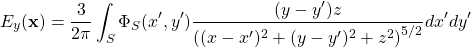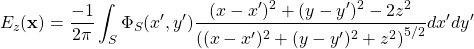where again we can view these as 2D convolutions. We also note that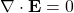can be shown for, as required.